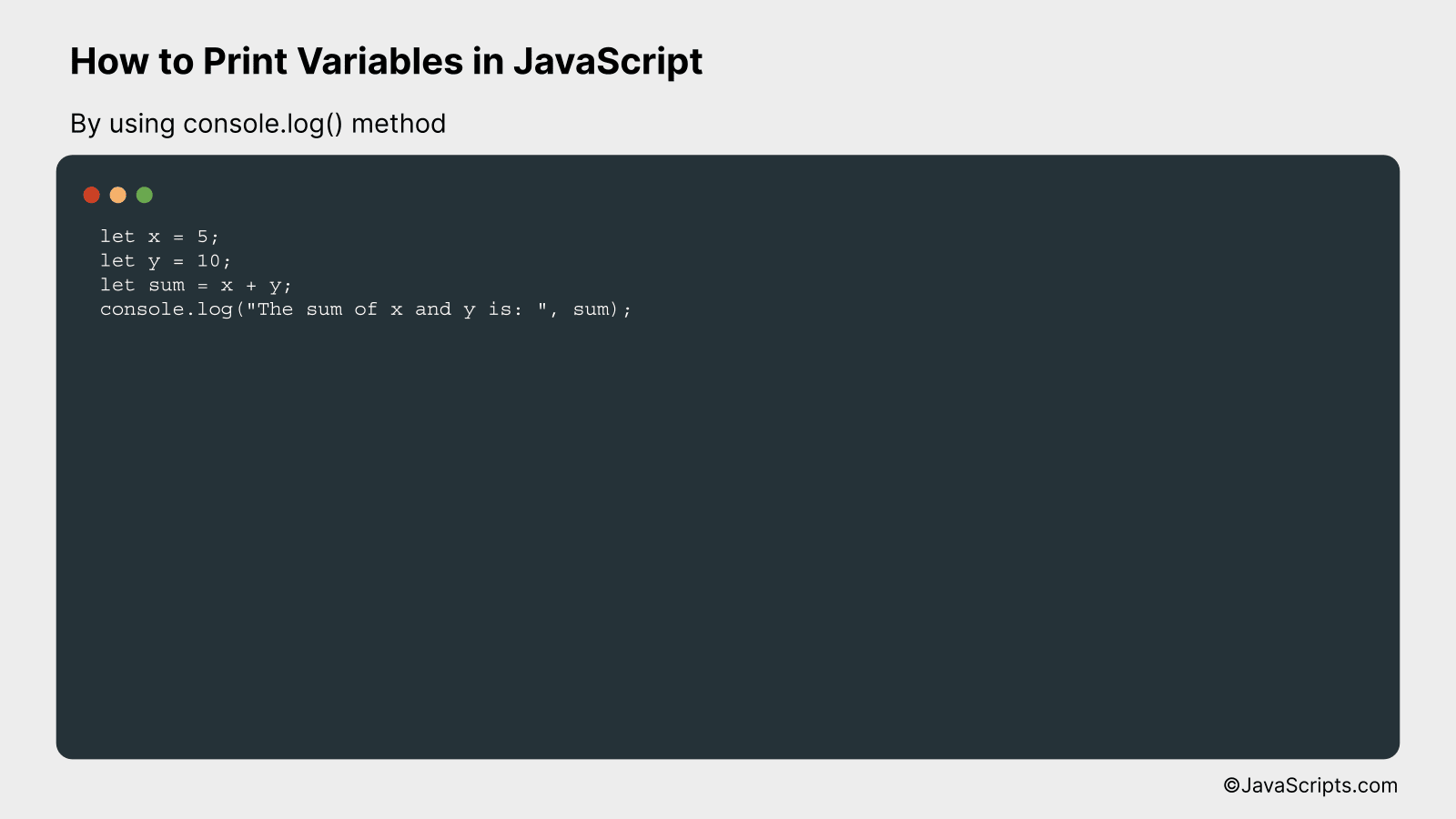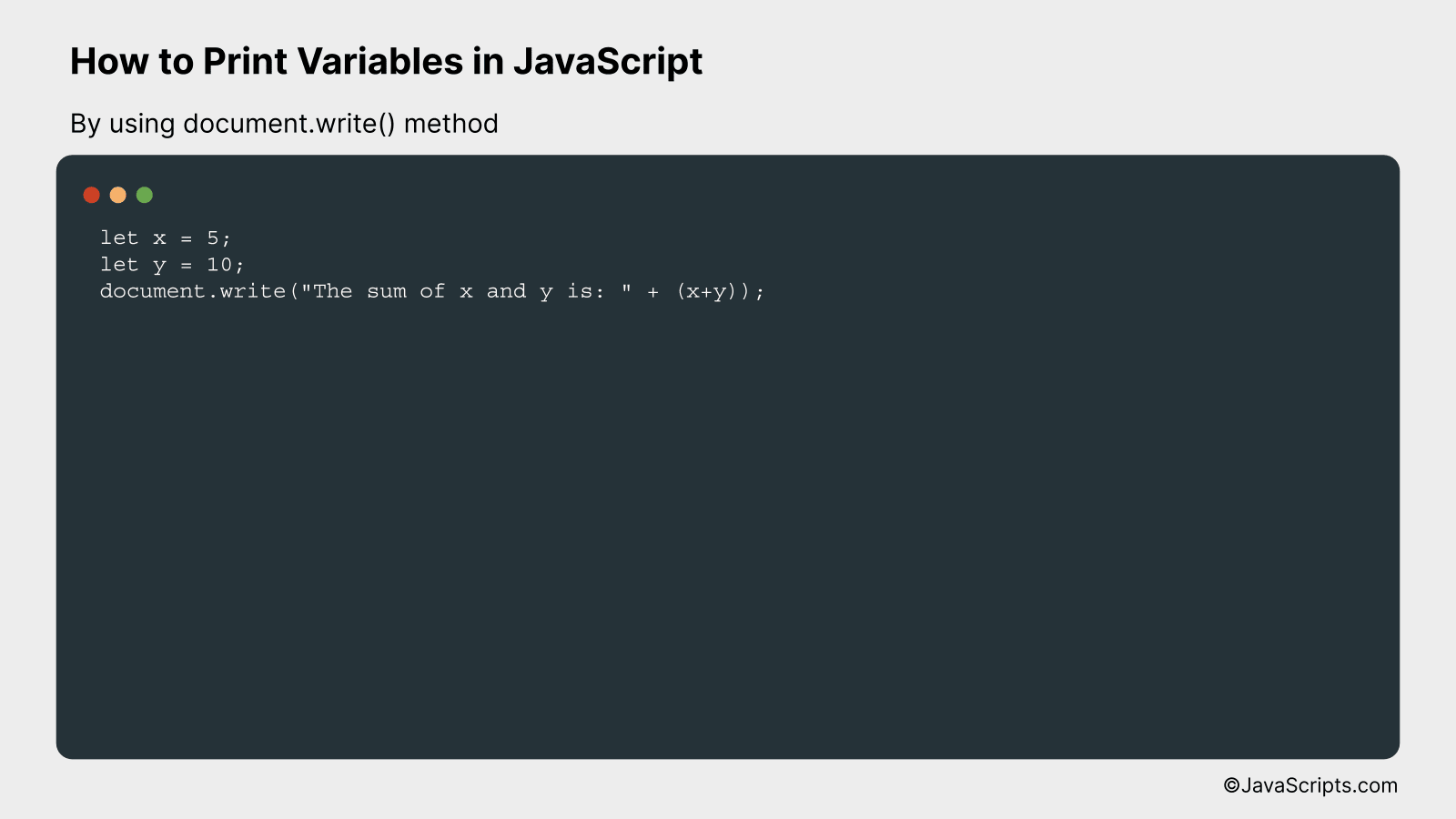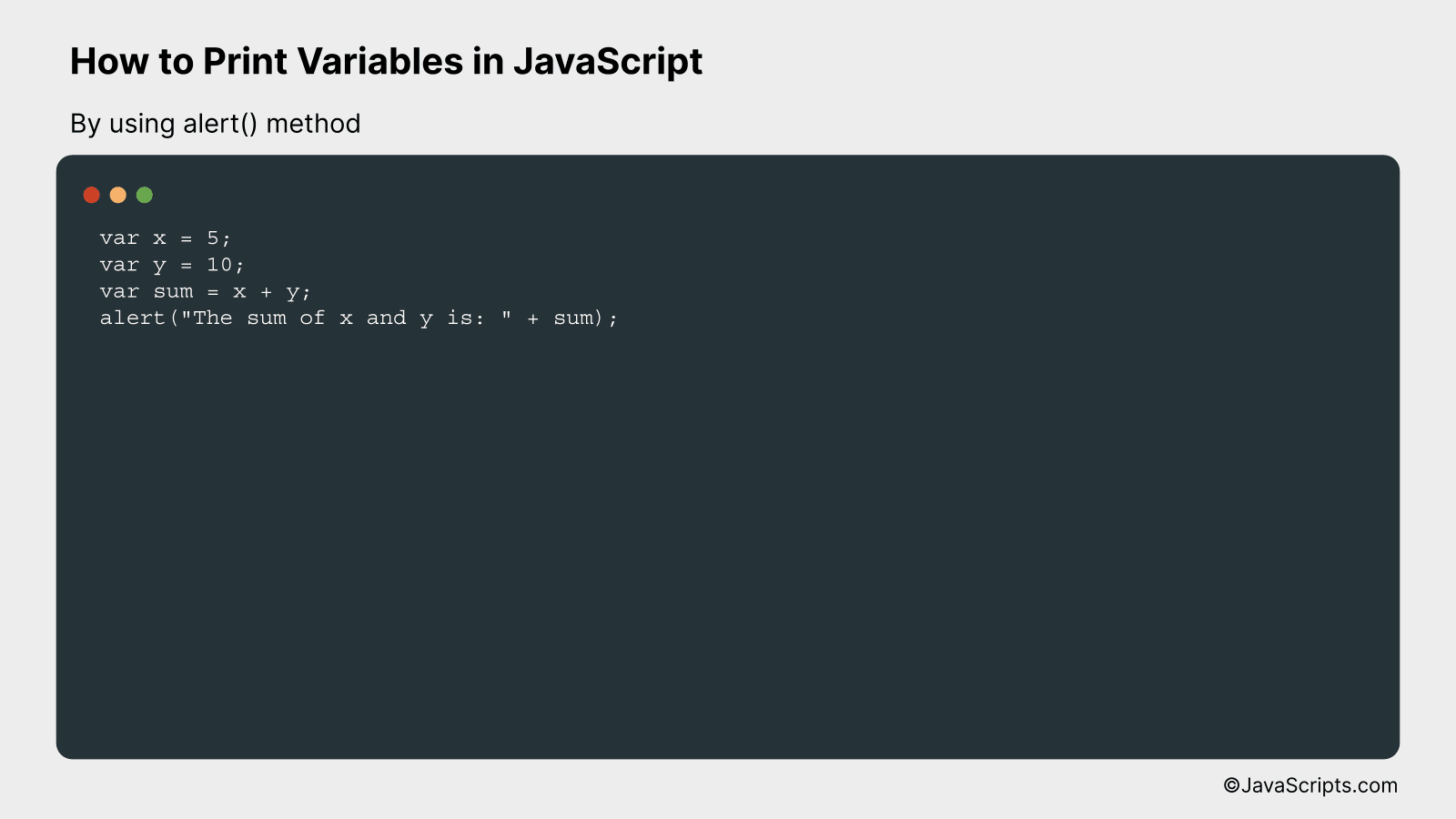# How to Print Variables in JavaScript

Welcome to the exciting world of JavaScript! As one of the fundamental building blocks of web development, JavaScript can feel a bit overwhelming at first. But don’t worry, we’re here to simplify it for you.

Let’s start by understanding a common task – printing variables. This seems simple, right? Well, it’s as easy as it sounds. But before diving in, let’s make sure we’re on the same page about what a variable is. Ready? Let’s begin.

In this post, we will be looking at the following 3 ways to print variables in JavaScript:

• By using console.log() method
• By using document.write() method

Let’s explore each…

## #1 – By using console.log() methodIn JavaScript, the console.log() method is used to print or display data in the browser’s console. This method is very useful for debugging purposes by logging any kind of variables, text, or other data types directly to the browser console.

``````
let x = 5;
let y = 10;
let sum = x + y;
console.log("The sum of x and y is: ", sum);
``````

### How it works

In the provided JavaScript code, we first declare and initialize two variables ‘x’ and ‘y’. We then compute their sum and store it in the variable ‘sum’. Finally, we use the console.log() method to print a message and the result of the addition to the browser console.

• First, we declare the variables ‘x’ and ‘y’ and assign them the values 5 and 10 respectively with the lines: let x = 5; and let y = 10;
• Next, we add the values of ‘x’ and ‘y’ and store the result in the variable ‘sum’ with the line: let sum = x + y;
• Finally, we print the message “The sum of x and y is: ” followed by the value of ‘sum’ to the console with the line: console.log(“The sum of x and y is: “, sum); This will print the message and the sum to the browser console.

## #2 – By using document.write() methodThe document.write() method in JavaScript is used to write content to a document. This method can be used to print variables in JavaScript, as shown in the following example:

``````
let x = 5;
let y = 10;
document.write("The sum of x and y is: " + (x+y));
``````

### How it works

The document.write() method in JavaScript writes a string of text into a document stream opened by document.open(). In this example, it is used to output the sum of two variables, x and y.

• The script tag is used in HTML to contain client-side JavaScript code or to reference an external JavaScript file.
• Variables x and y are declared and initialized to 5 and 10, respectively.
• The document.write() method is called and a string is passed as its argument. This string is a combination of a text string and the result of the addition of x and y.
• The browser will interpret and render this JavaScript code, and the output will be displayed on the webpage as: “The sum of x and y is: 15”.

## #3 – By using alert() methodThe alert() method in JavaScript displays an alert box with a specified message and an OK button. This method can be used to print variable values to understand their current state at a specific time. Here’s a simple example:

``````
var x = 5;
var y = 10;
var sum = x + y;
alert("The sum of x and y is: " + sum);
``````

### How it works

This code declares two variables ‘x’ and ‘y’, calculates their sum, and then displays it in an alert box using the alert() method.

• First, two variables ‘x’ and ‘y’ are declared and assigned values 5 and 10 respectively.
• Then, a new variable ‘sum’ is declared to store the result of the addition of ‘x’ and ‘y’.
• Finally, the sum of ‘x’ and ‘y’ is displayed in an alert box. The ‘+’ operator is used to concatenate the string and the sum variable.

Related:

In conclusion, printing variables in JavaScript is a simple yet important skill for every coder. It allows us to debug our code effectively, providing insights into how our program functions at every stage.

Remember, the console.log() function is your best friend in JavaScript for printing variable values. With it, you can easily keep track of your variables and how they change as your code runs. Keep practicing and happy coding!